0
2314In the past article, we have talked about the Arithmetic, Assignment, Increment/Decrement, and Range operators. In this article, we are going to complete our talk about operators.

Comparison Operators
Now, a very important type of operators: The Comparison operators. Comparison operators are essential for decision making. They compare numeric values, and return true if the condition is met. The following table lists the supported comparison operators in Perl:

 Operator Description == Equality operators. It checks whether the left side operand is equal to the right side one. < Less than. It checks whether the left side operand is less than the right side one. <= Less than or Equal. It checks whether the left side operand is less than or equal to the right side operand. > Greater than. It checks whether the left side operand is greater than the right side one. >= Greater than or equal. It returns true if the left side operand is greater than or equal to the right side one. != Inequality operator. It returns true if the two operands are not equal. <=> The complex comparison operator. It checks its two operands and returns: -1     if the left side operand is less than the right side one. 0      if both operands are equal. 1      if the left side operand is greater than the right side one.

Example
The following script will accept two numbers from the user. The script should compare the two numbers, and print the result of the comparison.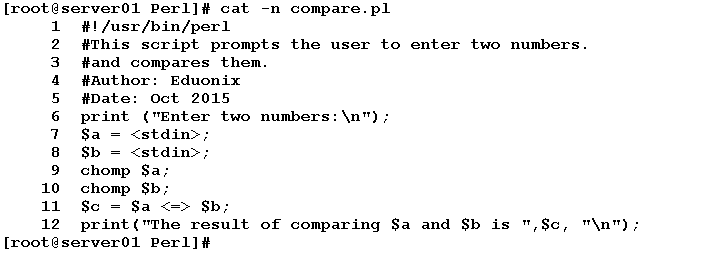Let’s see how this script will behave when executed: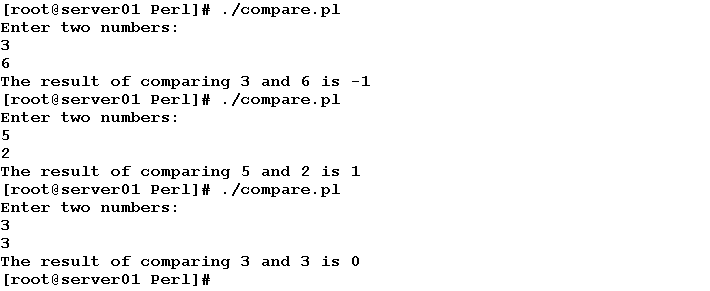Logical Operators
If you have studied Logic, you may have some knowledge of the Logic gates. There are three basic logic gates: AND, OR, and NOT. Consider two electrical switches connected together in series. For the current to flow from point to another across the two switches, both switches need to be ON. If one of them is OFF, the current won’t flow, and the result will be OFF. On the other hand, if the switches were in parallel, for the current to flow, only one switch needs to be ON. The third gate: the NOT gate inverts its input.

This is the concept of the logic gates in brief. The Logical operators do this job in software programs. The following table lists the logical operators supported in Perl:

 Operator Description And && The Logical AND operator. It returns true if (and only if) both operands are true. Or || The Logical OR operator. This operator returns true if either if its operands are ture. -= The Logical NOT operator. It inverses its input.

Example

The following script outputs true if the user’s input is greater than 0 and less than or equal to 10.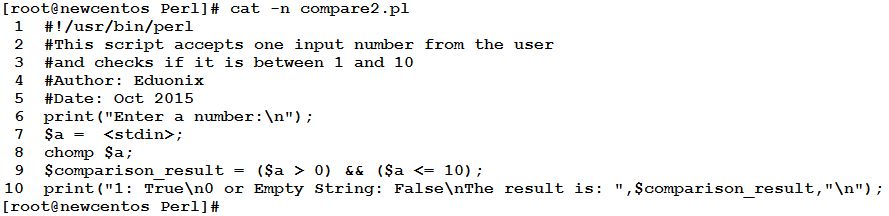Let’s see it in action: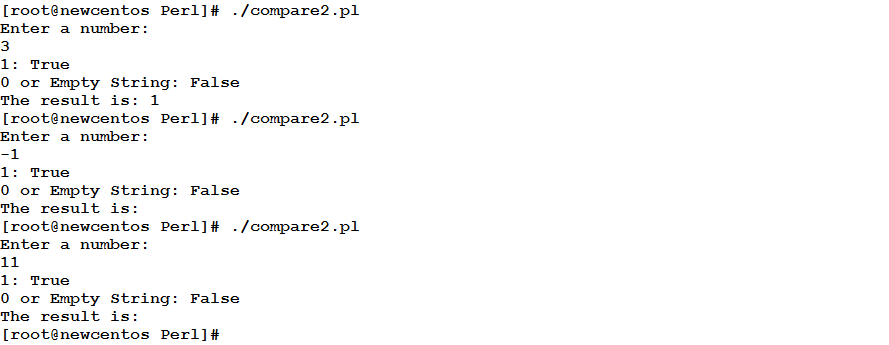Line 9:

• checks the entered number (which is stored in the variable \$a) if it is greater than 0
• checks if the entered number is less than or equal to 10
• performs a logical AND between the results of the two above comparisons.
• The true value in Perl can be represented by 1 (or non-zero value), while the empty string “” represents the false value.

String Operators
For strings concatenation and repetition, there are two operators:

• The Concatenation operator “.”

This operator concatenates (connects) two strings together to form a larger string.

• The String Repetition Operator “x”

This operator takes two operands: the first is the string to repeat, and the other is the number of times to repeat the provided string. The result of the operation is a string that consists of repeating the first operand (string) the specified number of times.Example
In this example, we are going to write a script that accepts the first name and the last name (as two separate inputs) from the user, and prints the full name.

Consider the following code: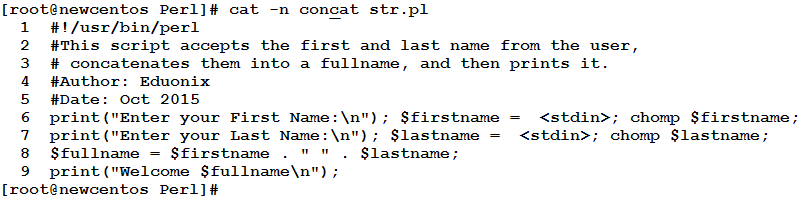Let’s see what we will get when we execute it:Line 8: uses the concatenation operator “.” to concatenate the string variable \$firstname, a white space, and \$lastname. The result of the concatenation is then assigned to the variable \$fullname.

Example
The following statement will create a string of “=” (could be used in formatting a table-like output)

`\$str_line = "=" x 30;`

When printed using the print function, we get the following output:

==============================

String Comparison Operators
As with numbers, Strings also have their comparison operators. I will mentioned here the two most important operators of this type: Equality and Inequality operators.

String Equality Operator eq
The string equality operator returns true if both operands (strings) are exact match.
Syntax

`(\$str1 eq #str2)`

String Inequality Operator ne
This is the opposite of the String Equality operator “eq”. It returns true if the left side operand is NOT equal to the right side one.
Syntax

`(\$str1 ne #str2)`

Example
Consider:

`\$country = "India";`

The following comparison will return false.

`(\$country eq "Brazil")`

On the other hand, this expression will return true:

`(\$country ne "india")`

Note
Notice that “India” doesn’t match “India”. That is why the inequality operator returns true when comparing them. The reason is clear, Perl is case sensitive.

* * * * * *

Summary